# Principle of relativity & generation and definition of force

вбга 561 ДЮдФЖС 2023-5-27 03:07 |ИіШЫЗжРр:ЮяРэбЇЁЂСІбЇМАздШЛембЇ|ЯЕЭГЗжРр:ПЦбаБЪМЧ

Keying Guan

Department of Mathematics, School of Science, Beijing Jiaotong University

Email keying.guan@gmail.com

Keying Guan

Department of Mathematics, School of Science, Beijing Jiaotong University

Email keying.guan@gmail.com

Abstract: The relation shared by Newtonian mechanics and relativistic mechanics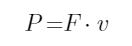(*)

violates the principle of relativity, leading to the fallacy that static force does not perform work. The relationship between power and its intrinsic force is in accordance with the principle of relativity. Using this relationship can clarify and redefine the principle of force generation. Newton's definition of force, especially the "inherent force of matter" (Inherent force of matter) contains profound ideas that are generally ignored.

Contents: 1. The principle of relativity and related issues

2. The definition and generation mechanism of force

3. The Natural Philosophy embedded in the combination of Newton and Einstein

1. The principle of relativity and related issues

The principle of relativity is a universal principle for human beings to describe the objectivity of natural phenomena. Generally speaking, the principle of relativity requires that objective natural phenomena or laws have nothing to do with the space-time frame (generally referred to as the inertial frame) chosen by the observer. Modern physics regards the principle of relativity as the basic principle of physics (refer to , , , , ). Especially Einstein's paper  entitled "On the Principle of Relativity and Its Derived Conclusions" (Über das Relativitätsprinzip und die aus demselben gezogenen Folgerungen), based on the principle of relativity, deduced the mechanics of special relativity (including the electromagnetic science, motion systems, and thermodynamics (such as entropy and temperature)).

Newtonian mechanics and special relativity have the same requirements on the inertial system, that is, objects that move freely without external force in the inertial system must move in a straight line at a uniform speed.

The space-time used by Newtonian mechanics is Galilean space-time. Time and space coordinates are separated from each other and measured according to their own standards, and the speed of motion satisfies the principle of linear superposition, so there is no upper limit to the speed of objects in nature.

The mass m of an object moving at a constant speed v in Newtonian mechanics is a well-known physical invariant, but the kinetic energy  K (=1/2 mv2) and momentum p (= mv) obviously do not comply with the principle of relativity.

The special theory of relativity takes the invariance of the speed of light as the basic principle, so the space-time of the inertial system can only reasonably adopt the Minkowski space-time, and the transformation between the inertial systems can only be carried out according to the relationship of Lorentz transformation. In special relativity, although some objective physical quantities are related to relative velocities in different inertial systems, they must be transformed according to the covariant requirements of Lorentz transformation. Such as energy, mass, four-dimensional acceleration, four-dimensional force, four-dimensional potential of electromagnetic field, energy-momentum tensor, electromagnetic field tensor and so on.

But in the special theory of relativity, there are also physical quantities that do not depend on the inertial system, such as the interval between events, the proper time, the rest mass m0 of particles , and the electric charge e of electrons , etc. are all invariants. In addition, the two physical quantities E2 - H2, and EЁёH of the electromagnetic field are also invariants, where E and H are the electric field strength and magnetic field strength respectively.

The most important invariant relation in the special theory of relativity is the equivalence relation between the object energy E and mass  m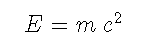(1)

where c is the speed of light. This relationship is the greatest contribution of special relativity to human understanding of the material world.

Equation (1) is also equivalent to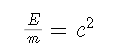(1')

That is to say, the ratio of energy to mass of matter is an invariant.

Interestingly, in Newtonian mechanics, Newton's second law means that the force   is equal to the mass of the object under force times the acceleration  a ЃЌ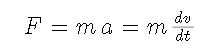(2)

Obviously, acceleration is also a quantity independent of velocity. Therefore, in Newtonian mechanics, both mass   and force  F   are invariants that have nothing to do with the choice of inertial system.

Another interesting phenomenon is that in Newtonian mechanics and special relativity, Power  P  is defined as the rate of change of energy  E  versus time, that is,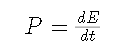(3)

Similarly, according to theoretical physics theory, force  F is defined as the time rate of change of momentum  p (or impulse)  in both Newtonian mechanics and special relativity ,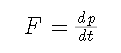(4)

For power P and force F , both Newtonian mechanics and special relativity can derive the same relationship, that is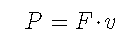(5)

In Newtonian mechanics, since the energy E of a freely moving object is equal to its kinetic energy K , formula (5) can be directly proved.

The author mentioned many times in previous blog posts that Einstein had already used the relation (5) in his first paper on the creation of the special theory of relativity. However, since the theory was just proposed at that time, the paper did not give Equation (5) conforms to the derivation of the theory of relativity, and in 1906, the German physicist M. Planck (Max Planck) had already stated for the first time that the description (or definition) of force in this paper was not the most favorable. Planck pointed out: A more pertinent definition of force should make the law of momentum and law of energy have the simplest form. This fact can be found in the note  on page 63 of  (or the note on page 112 of ), which was given by the famous German theoretical physicist A. Sommerfeld.

In fact, since the 20th century, several top physicists have generally used the principle of least action (or Hamiltonian principle) in their systematic works on theoretical physics to describe Newtonian mechanics, special relativity, general relativity, and even quantum mechanics, for example, the works of Planck , the works of Landau and Lipschitz , , , the works of S. Weinberg (Stevev Weinberg)  This is true.

In order to save space, this paper omits the introduction of the minimum action and the basic concepts of relativity, and directly uses Landau and Lipschitz's <The Classical Theory of Fields> , in the simplest case, the following The process of deriving the formula (5) based on the principle of least action.

Suppose a particle with a constant rest mass m0 in vacuum   moves with a constant velocity v  relative to a stationary observer  .

The three-dimensional velocity  v  is expressed in ordinary space Cartesian coordinates as,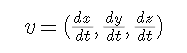According to the principle of least action in the theory of relativity, to describe the motion of a particle, a basic invariant to describe the motion of a particle under the Lorentz transformation is the interval generated by the instantaneous motion of particles in the four-dimensional Minkowski space-time(6)

The action S of particle motion established on the basis of this invariant can be expressed as(7)

where the Lagrange function  L  is(8)

According to the definition, the momentum vector  p of particle  m0   is(9)

Similarly, according to the definition, the energy of particle  m0  is(10)

In special relativity, due to the following two factors often appearTherefore, for the sake of simplicity, they are generally recorded as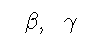make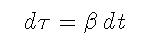And accordingly define  Іг  as the intrinsic time of the particle. Obviously, if the velocity of the particle is zero, the intrinsic time is synchronized with ordinary time.

From (9) and (10), we can see that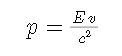(11)

According to the definition, since the four-dimensional inverse velocity of particle  m0  is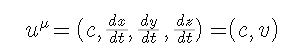(12)

The corresponding covariant four-dimensional velocity is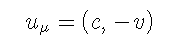(13)

The four-dimensional inverse motion of a particle is defined as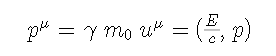(14)

Then, the four-dimensional covariation of the particle is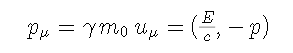(15)

The inverse form of the particle  0  subject to the four-dimensional force is defined as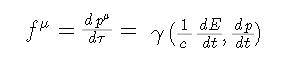(16)

According to formulas (9) and (10), it can be calculated that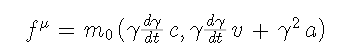(17)

in which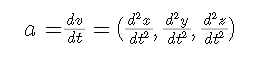(18)

It is the ordinary acceleration of particles in space. The covariant form of the four-dimensional force is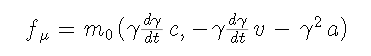(19)

can be calculated directly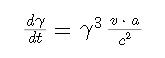(20)

The spatial part of the four-dimensional force is denoted as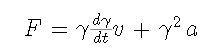(21)

It is not difficult to see that when the velocity v of the particle is relatively small relative to the speed of light, the space part of the above four-dimensional force differs very little from the force of Newtonian mechanics, especially when v = 0 , F is exactly the same as Newtonian force.

Substituting formula (20) into formula (19), further calculation can find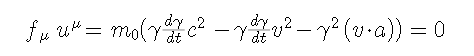(22)

Since the covariant form of the four-degree force can be written as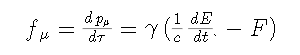(23)

(22) means that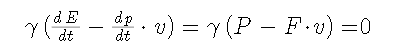(24)

Obviously (24) is equivalent to (5).

In a slightly complicated situation, for example, when there is an electromagnetic field, if a particle with a static mass of m0 and a constant charge is not enough to significantly affect the surrounding electromagnetic field, then the particle will suffer in the electromagnetic field Lorentz force of electromagnetic field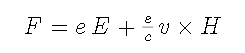(25)

In this case, it can still be proved (the proof is omitted in this paper, and the detailed proof can refer to )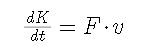(26}

where K is the kinetic energy of the particleIf dK/dt is understood as the power P of charged particles, then (26) is equivalent to (5).

Therefore, formula (5) can also be derived in special relativity mechanics.

The above discussion about the principle of relativity still stays within the framework of Newtonian mechanics and special relativity. When extended to include general relativity, which includes universal gravitation, general relativity is embodied in Einstein's "Principle of Equivalence of Gravity and Inertia". To keep this principle valid on a large scale requires the assumption that spacetime is curved by the presence of matter, which requires non-Euclidean geometry of curved spacetime, using Christopher's notation and many forms of complex covariant tensors . This paper omits the description of these complex details, just pointing out that relational expressions with the same essence as (5) also exist. Readers interested in details are referred to  or .

Since the relation  and each quantity in it are obviously related to the choice of the inertial frame where the observer is located, it obviously does not satisfy the principle of relativity. This poses an important challenge to theoretical physics: that is, since the relational formula (5) does not conform to the principle of relativity, is there a problem of objectivity in this formula?

In fact, formula (5) can also be written as (note, the vector division commonly used in physics is used)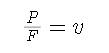(5')

It can be seen that the formula (5) means that the static force does not do work, and the formula (5') means that the ratio of the force to the energy consumption rate to produce this force depends on the speed relative to the observer! This is obviously an absurd conclusion that goes against objective facts.

For example, the author saw that when the power department in California, USA erected an elevated transmission line, it needed to hang the device for a short period of time when it used a helicopter to send the remote device to the hands of the constructor on the elevated. During this period, the force supporting the gravity of the device is a static force (see Figure 1). Can the helicopter not consume energy for this? Is the gravity of the device and the energy consumption of the helicopter related to the observer?figure 1.

In addition, it is reported that in a certain flight, in order to maintain lift and overcome air resistance, a Boeing 787-9 flying at a constant speed burned about 5,400 liters of fuel per hour.Figure 2 (from 360 Encyclopedia entry: Boeing 787)

Could this fact be related to the speed of the observer relative to the plane? More examples that violate common sense are also given in the author's previous blog posts.

In fact, the internal relationship between correct response power and force has been implied in their definitions.

In the blog post  Power and its intrinsic force  2023-04-15ЃЌthe author pointed out that this intrinsic relationship has been reflected in any scientific and complete measurement system. The internal relationship between the power  P  and its intrinsic force  Fintrinsic  is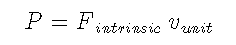(27)

or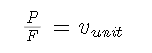(27')

where  vunit  is the unit velocity in the corresponding metric system. It is a quantity that has nothing to do with the choice of inertial frame. Therefore, the relation (27) is an objective relation completely irrelevant to the observer. This relationship is neither Newtonian mechanics, nor relativity mechanics, nor is it the derivation result of quantum mechanics, biomechanics, etc. If concepts such as energy, mass, force, and power are defined reasonably, and when they are measured with complete measurement rules, the profound equivalence relationship (27) or (27') between power and force must appear!

2. The definition and generation mechanism of force

The author thinks that the reason for the absurd conclusion brought by the above formula (5) or (5') is that the mainstream theoretical physics circles define the concept of force F according to formula (4). This definition depends on the momentum of the force recipient or force generator, but in static force, the momentum of both force generator and force recipient is equal to zero, so this definition is not suitable for static force.

Newton's gravitation, or the Lorentz force in the electromagnetic theory all exist objectively. Objects affected by this force may encounter external resistance and stop moving. At this time, although the gravitational force or the Lorentz force become the static force, and the resistance also becomes the static force, they still exist objectively. If the force defined by formula (4) is still required to satisfy formula (5), this will inevitably lead to the wrong conclusion that force does not do work. Therefore, there are major flaws or omissions in the definition of force in the mainstream theory.

In fact, Isaac Newton had already defined force as early as 1686 in his " Mathematical Principles of Natural Philosophy "Figure 3

In this work  , Newton started with definitions (conventions) and "axioms and laws", expounding universal gravitation and the three major laws of motion.

The definition part is on pages 403 to 415 of the book, and there are eight definitions: definition 1 "quantity of matter", definition 2 "quantity of motion", definition 3 "inherent force of matter" , definition 4 " Impressed force , Definition 5 "Centripetal force" , Definition 6 " The absolute quantity of centripetal force "  , Definition 7 " The acceleration quantity of centripetal force" and Definition 8 "The motive quantity of centripetal force".

These definitions are described in words. Limited to the understanding level of that era, it is fully understandable for Newton to divide force into six parts to define. Obviously, definitions 3 and 4 are the core parts:

Definition 3  Inherent force of matter is the power of resisting by which every body, so far as it is able, a perseveres in its state either of resting or of moving uniformly straight forward.

Definition 4  Impressed force is the action exerted on a body to change its state either of resting or of moving uniformly straight forward.

After giving the definition, Newton passed the axioms (Axioms of the laws of motion), that is, the famous Newton's three laws of motion to constrain the force exerted on the mechanically moving object. The second law is as follows:

Law 2   A change in motion is proportional to the motive force impressed and takes place along the straight line in which that the force is impressed.

Obviously, Newton did not directly define force through Newton's second law (formula (4)), but used the second law to explicitly constrain the " Impressed force" he defined . This fully shows that Newton believed that force is a potential objective existence.

When he used more words to describe the "inner force of matter", which he put at the top of the definition of force, he expressed it as follows:

This force is always proportional to the body and does not differ in any way from the inertia of the mass except in the manner in which it is conceived. Because of the inertia of matter, every body is only with difficulty put out of its state either of resting or of moving. Consequently, inherent force may also be called by the very significant name of force of inertia. Moreover, a body exerts this force only during a change of its state, caused by another force impressed upon it, and this exercise of force is, depending on the viewpoint, both resistance and impetus: resistance insofar as the body, in order to maintain its state, strives against the impressed force, and impetus insofar as the same body, yielding only with difficulty to the force of a Resisting obstacle, endeavors to change the state of that obstacle. Resistance is commonly attributed to resting bodies and impetus to moving bodies; but motion and rest, in the each other only by point rest are not always truly popular sense of the terms, are distinguished from of view, and bodies commonly regarded as being at rest .

This shows that the "internal force of matter" defined by Newton should include "static force"; it also shows that he was thinking and exploring for the formation of internal force.

If Newton had known the equivalence relationship between mass and energy at that time, then he would have given a deeper definition of the internal force of matter.

After understanding why Newton put the "inner force of matter" at the top of the definition of force, the author sincerely admires the depth of Newton's thought!

Strictly speaking, the mainstream definition of force (4) ignores the objective fact that power and its intrinsic force are produced at the same time and at the same place, ignores the equivalence relationship between power and intrinsic force contained in the scientific unit system, and ignores the power and force generation essence.

In fact, the equivalence relationship between power and force has long been contained in human cognition (or consciousness). In the Magdeburg hemisphere experiment, people used horse power to measure the force provided by the horse in the experiment, and people also more or less The word horsepower is rarely used consciously as a measure of power magnitude. In particular, the word power has dual meanings of power and force in many languages. According to this relationship, the power consumed by a horse is equivalent to the internal force output by the horse, and the power consumed by the engine in one watt is equivalent to the internal force output by the engine in one Newton.

The following discusses the relationship between the intrinsic force intrinsic and the externally visible and directly felt force F.

If within a period of time, the power P in the formula (5), the force F that can be felt, and the non-zero velocity v are all constant values, which means that the force generator must move at the velocity v during this period, and is subject to some resistance of intensity equal to F. At this time, the relationship between F and the internal force Fintrinsic of the effective power P issued by the generator is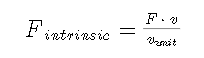(28)

For this reason, the force generator needs to transform the given intrinsic force intrinsic into a given force F through gears, levers, pulleys and other structures.

If the force F is a pure static force, and no matter how great the static force is, the object receiving the force cannot produce direct macroscopic motion, then the thrust F of the force generator is equal to the reaction force of the object receiving the force. At this time, the power sent by the force generator must be equal to the power sent by the force receiver to the force generator, and the energy transmitted by the force generator to the force receiver within a short period of time must be equal to the energy transmitted by the force receiver to the force generator. This fact means that in the case of static force, the static force is equal to the internal force emanating from the force generator.

If the object receiving the force does not experience any motion resistance, then the formula (5) is meaningless, and the object acting on the force F must do accelerated motion. Assuming that the moving speed of the object before the force is equal to zero, as pointed out in the previous section, at the moment when the force F starts to act, both Newtonian mechanics and special relativity mechanics are equal to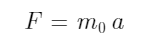(2ЁЏ)

where m0 is the rest mass of the object under the force and a is the acceleration of the object. At this moment of action, the force F can be regarded as a static force, so F = Fintrinsic. If the force-bearing object has been moving at a certain speed v, according to the special theory of relativity, formula (2') must be modified into formula (21). If the giver always follows the receiver, there must also be F = Fintrinsic.

No matter what kind of given force F can be felt, the force-applier can make the internal force Fintrinsic appear as the given force F instantly through the control of the auxiliary structure, just like Archimedes imagined when studying the lever The: "Give me a fulcrum, I can shovel the earth."Figure 4 (The picture is taken from the Chinese Wikipedia entry: Archimedes)

Indeed, if conditions permit, Archimedes can indeed provide such a great power in an instant. But if you really want to move the earth, it takes time and you need to really put in physical energy, which was very difficult for Archimedes. In fact, as long as there is enough energy, a small internal force can overcome a lot of resistance. For example, with tools such as pulleys, people with little strength can still lift heavy objects.

It can be seen from the above that the equivalence between force and internal force really gives the root cause of force.

According to these facts, the author thinks that it may be more natural and scientific to use internal force as a general definition of force including static force. Theoretically, if the principle of least action is used, after the definition of energy and momentum, power and internal force can be defined first, that is, to reveal the source of force generation, and then define the force acting on the moving object, so that the theory will be more complete and have more Great adaptability.

Clearly, the activation of energy comes from two aspects:

On the one hand, it comes from the inside of the object. In other words, if its internal energy (see the note 1) is unstable and undergoes internal changes over time, the internal energy is activated, that is, the internal energy changes with time to generate power and internal force;

Note 1: The definition of internal energy can refer to  and 

On the other hand, the particle is subjected to the contact force brought by other substances, or the effect of the electromagnetic field and the gravitational field, etc.

The generation of force is accompanied by the interaction of matter and the transformation of energy form.

In modern theoretical physics, such as the gravitational interaction between particles in Newtonian mechanics, the interaction between charged particles and electromagnetic fields in special relativity, and the interaction between matter and space-time geometry in general relativity, etc., all partially reflect energy and internal forces. Activation mechanisms, such as radiation mechanisms of electromagnetic fields. In addition, issues involving quantum mechanics, quantum field theory, elementary particles, cosmology and various aspects of natural science are also important research objects.

These theories are developing and have achieved much, but they also have inherent difficulties that need to be explored and refined. For example, regarding elementary particles with rest mass (including electrons above all), do they exist as purely geometric moving points in space-time; do they have internal structure; how do they interact with space-time; since they have mass, they have internal Energy, whether their internal energy can be stimulated or can be stimulated by themselves, etc.

3. The Natural Philosophy Embedded in the Combination of Newton and Einstein

Physics is the basic science that studies the material world. The author believes that the energy E and the rate of change of energy (namely power) P are the most basic quantities in physics to describe matter and matter motion (including interaction). According to the observer-independent equivalence relation between energy and mass (3) and the equivalence relation between power and intrinsic force (27), we can define mass and force, which are important concepts in physics to describe the world.

Power and internal force are the manifestations of activated energy. Without this activation, the entire material world is a cold, lifeless, dead world. Therefore, the study of energy activation is a major task in physics research.

There is a porcelain coffee cup in front of the author, which is very strong and has a beautiful shape. Is its inner energy alive? Think about it and realize that it is alive. Why? Because this cup is very strong, it means that its constituent substances have strong binding force, and if there is binding force, the force will do work, and the internal structure will continue to change. From this, I thought that no matter how carefully maintained, this coffee cup will definitely change into another form of existence.

Therefore, this world is alive and all matter is alive.

The Newton's profound thoughts on  "internal force of matter", Einstein's theory of relativity and the equivalence relationship between energy and mass revealed by it are all great ideological wealth of mankind.

The equivalence relationship between energy and mass, and the equivalence relationship between power and its internal force are the most important relationships in nature, and the two complement each other and depend on each other.

These two equivalences conform to the more general principle of general relativity that they exist independently and objectively for any human observer in any situation.

references:

  Einstein, Albert (1905d) [Manuscript received: 30 June 1905]. Written at Berne, Switzerland. "Zur Elektrodynamik bewegter Körper".  Annalen der Physik  (Submitted manuscript) (in German). Hoboken, New Jersey (published 10 March 2006).  322  (10): 891ЈC921.

  Einstein, Albert (1907),  Über das Relativitätsprinzip und die aus demselben gezogenen Folgerungen. Jahrbuch der Radioaktivität under Eleltronik, 1907, V 4, 411-462

 MAX PLANCK, EIGHT LECTURES ON THEORETICAL PHYSICS, DELIVERED AT COLUMBIA UNIVERSITY IN 1909, TRANSLATED BY AP WILLS, PRESS OF THE NEW ERA PRINTING COMPANY LANCASTER, PA. 1915

  Landau , Lev D.; Lifshitz, Evgeny M. (1976).  Mechanics . Vol. 1 (3rd ed.). Butterworth-Heinemann. ISBN   978-0-7506-2896-9 _.

  andau, Lev D.; Lifshitz, Evgeny M, THE CLASSICAL THEORY OF FIELDS , Translated from the Russian by MORTON HAMERMESH, PERGAMON PRESS OXFORD • NEW YORK • TORONTO SYDNEY ' BRAUNSCHWEIG , Third English edition 1971

   andau, Lev D.; Lifshitz, Evgeny M,  QUANTUM MECHANICS, Translated from the Russian by JB SYKES and JS BELL, PERGAMON PRESS Member of Maxwell Macmillan Pergamon Publishing Corporation OXFORD NEW YORK BEIJING FRANKFURT SAO PAULO • SYDNEY • TOKYO • TORONTO , Third revised edition 1977.

 Steven Weinberg,  Gravitation and Cosmology: Principles and Applications of the General Theory of Relativity, by Steven Weinberg, pp. 688. ISBN 0-471-92567-5. Wiley-VCH , July 1972.

 HA Lorentz, A. Einstein, H. Minkowski and L. Weyl, The Principle of Relativity,  Dover Publications (June 1, 1952)

  Compiled by Fan Dainian, Zhao Zhongli and Xu Liangying, Collected Works of Einstein, Volume II, published by Commercial Press, first edition in 1977, unified ISBN: 2017 184

 Isaac Newton, THE PRINCPIA Mathematical Principles of Natural Philosophy, a new translation and guide by I. Bernard Cohen and Anne Whitman associated by Julua Budenz, University of California Press, Berkeley, Las Angeles, Landon, 1999

https://blog.sciencenet.cn/blog-553379-1389152.html

ЩЯвЛЦЊЃКЯрЖдадд­РэКЭСІЕФВњЩњгыЖЈвх
ЪеВи IP: 99.8.68.*| ШШЖШ|

### ИУВЉЮФдЪаэзЂВсгУЛЇЦРТл ЧыЕуЛїЕЧТМ ЦРТл (0 ИіЦРТл)

Ъ§ОнМгдижа...
ЩЈвЛЩЈЃЌЗжЯэДЫВЉЮФ

## ШЋВПОЋбЁВЉЮФЕМЖС

GMT+8, 2023-10-4 01:56